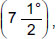# User Forum

Subject :IMO    Class : Class 7

Which of the following statements is INCORRECT?

A If length of any two sides of a triangle are 7 cm and 10 cm, then length of its third side lies between 3 cm and 17 cm.
B It is possible to construct a unique triangle if all its three angles are given.
C An angle ofcan't be constructed using compasses and ruler.
D None of these

## Ans 1:

Class : Class 5
The option b states that a unique triangle is constructed and it is possible to do so. However option c states that we CANNOT construct the angle 7.5. This is false cause it can be constructed by bisecting an angle of 60, three times.

Class : Class 7

Class : Class 7
I mean B

## Ans 4:

Class : Class 8
both B and C are correct as angle 7.5 can be constructed using compass and ruler and we cant construct a unique triangle with only 3 angles given.

## Ans 5:

Class : Class 8
ya guys the answer is D

## Ans 6:

Class : Class 9
Aditya that is the very reason why it will be a unique triangle and option C is correct since it an angle such as that can be created by bisecting 60 degree three times

## Ans 7:

Class : Class 7
It is meant to be c

Class : Class 8

Class : Class 8

## Ans 10:

Class : Class 8
ans is b.i agree with aditya

## Ans 11:

Class : Class 8
Answer is b as the length of sides of triangle are variable and therefore unique triangle cannot be constructed

Class : Class 7

Class : Class 8